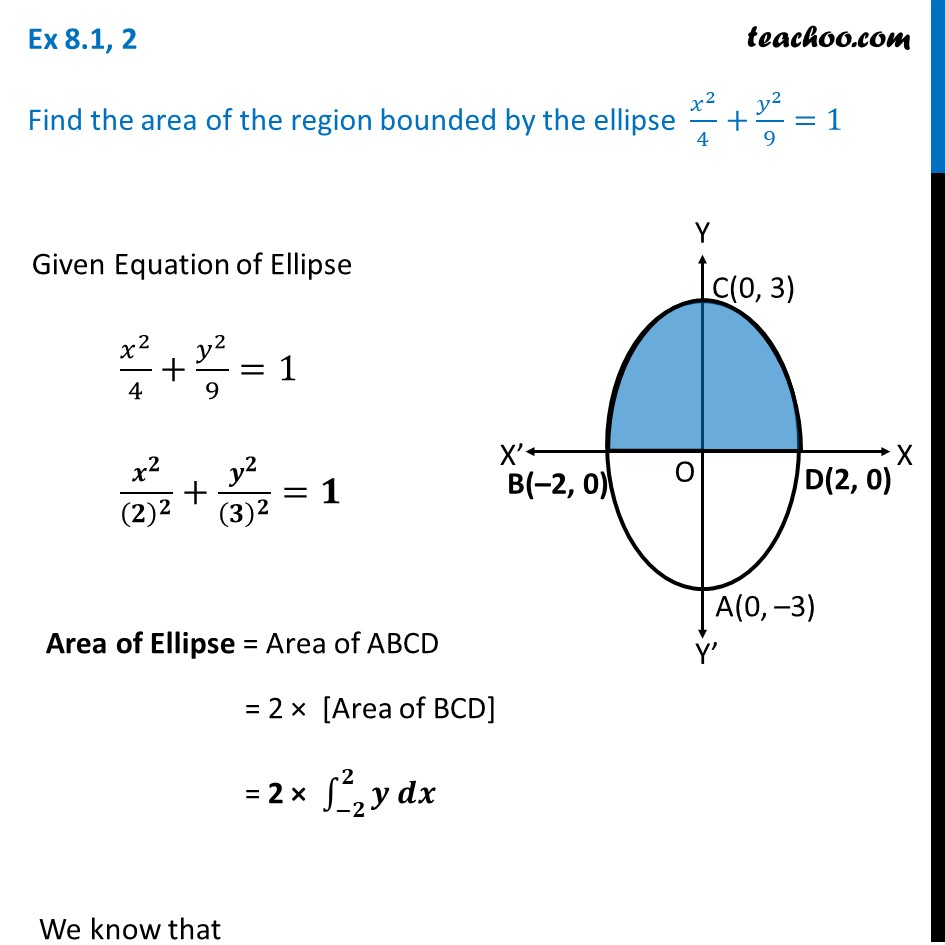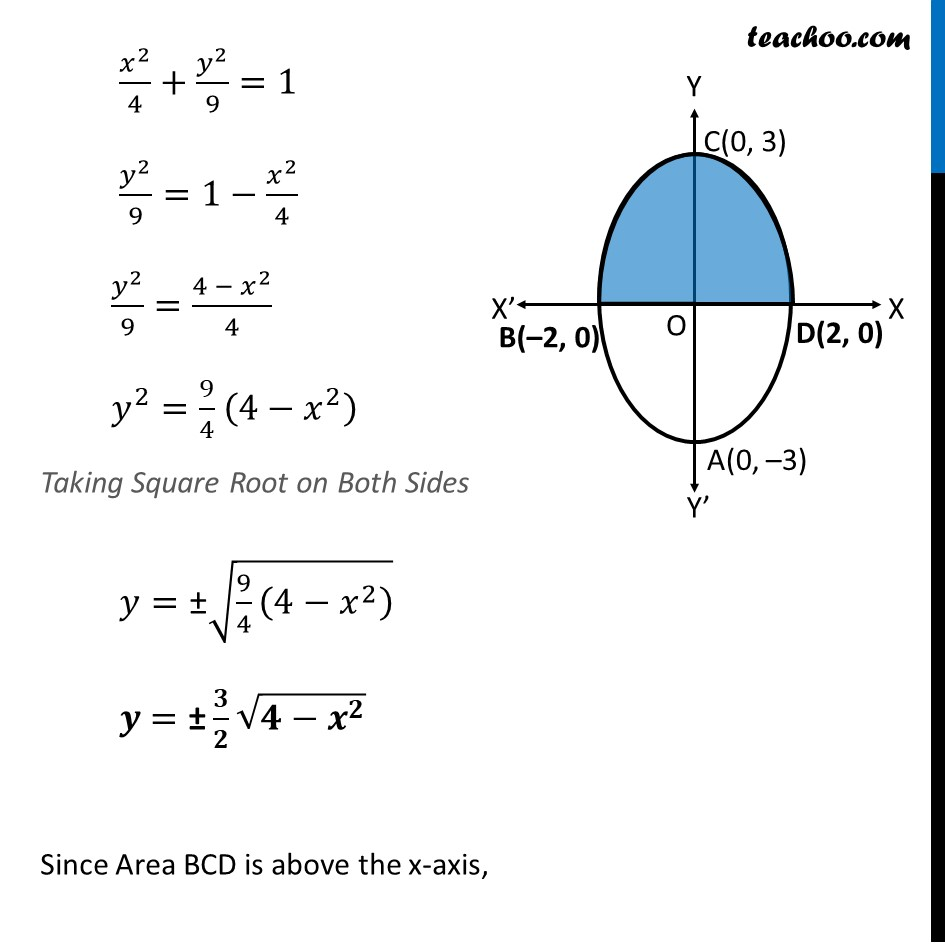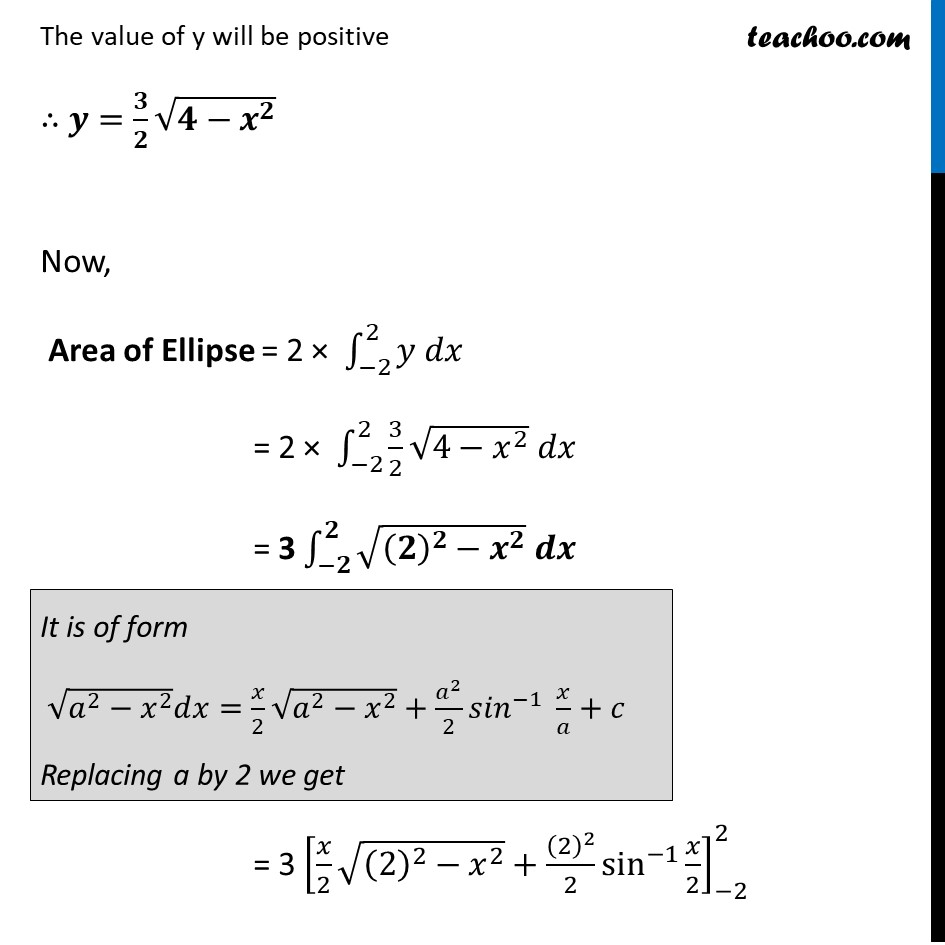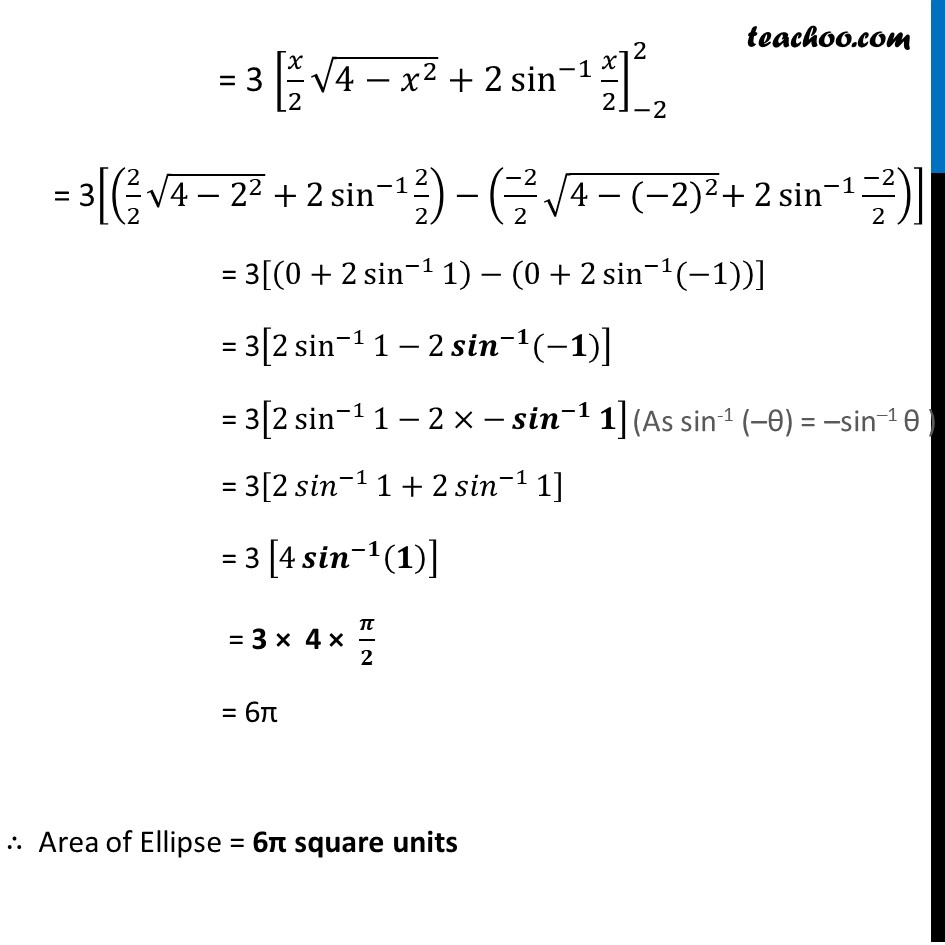Ex 8.1

Chapter 8 Class 12 Application of Integrals
Serial order wiseLearn in your speed, with individual attention - Teachoo Maths 1-on-1 Class

### Transcript

Ex 8.1, 2 Find the area of the region bounded by the ellipse 𝑥^2/4+𝑦^2/9=1Given Equation of Ellipse 𝑥^2/4+𝑦^2/9=1 𝒙^𝟐/(𝟐)^𝟐 +𝒚^𝟐/(𝟑)^𝟐 =𝟏 Area of Ellipse = Area of ABCD = 2 × [Area of BCD] = 2 × ∫_(−𝟐)^𝟐▒〖𝒚 𝒅𝒙〗 We know that 𝑥^2/4+𝑦^2/9=1 𝑦^2/9=1−𝑥^2/4 𝑦^2/9=(4 − 𝑥^2)/4 𝑦^2=9/4 (4−𝑥^2 ) Taking Square Root on Both Sides 𝑦="±" √(9/4 (4−𝑥^2 ) ) 𝒚 ="±" 𝟑/𝟐 √(𝟒−𝒙^𝟐 ) Since Area BCD is above the x-axis, The value of y will be positive ∴ 𝒚=𝟑/𝟐 √(𝟒−𝒙^𝟐 ) Now, Area of Ellipse = 2 × ∫_(−2)^2▒〖𝑦 𝑑𝑥〗 = 2 × ∫_(−2)^2▒〖3/2 √(4−𝑥^2 ) 𝑑𝑥〗 = 3 ∫_(−𝟐)^𝟐▒〖√((𝟐)^𝟐−𝒙^𝟐 ) 𝒅𝒙〗 It is of form √(𝑎^2−𝑥^2 ) 𝑑𝑥=𝑥/2 √(𝑎^2−𝑥^2 )+𝑎^2/2 〖𝑠𝑖𝑛〗^(−1)⁡〖 𝑥/𝑎+𝑐〗 Replacing a by 2 we get = 3 [𝑥/2 √((2)^2−𝑥^2 )+(2)^2/2 sin^(−1)⁡〖𝑥/2〗 ]_(−2)^2 = 3 [𝑥/2 √(4−𝑥^2 )+2 sin^(−1)⁡〖𝑥/2〗 ]_(−2)^2 = 3[(2/2 √(4−2^2 )+2 sin^(−1)⁡〖2/2〗 )−((−2)/2 √(4−〖(−2)〗^2 )+2 sin^(−1)⁡〖(−2)/2〗 )] = 3[(0+2 sin^(−1)⁡1 )−(0+2 sin^(−1)⁡〖(−1)〗 )] = 3[2 sin^(−1)⁡1−2 〖𝒔𝒊𝒏〗^(−𝟏)⁡〖(−𝟏)〗 ] = 3[2 sin^(−1)⁡1−2×−〖𝒔𝒊𝒏〗^(−𝟏)⁡𝟏 ] = 3[2 〖𝑠𝑖𝑛〗^(−1)⁡1+2 〖𝑠𝑖𝑛〗^(−1)⁡1 ] = 3 [4 〖𝒔𝒊𝒏〗^(−𝟏)⁡(𝟏) ] = 3 × 4 × 𝝅/𝟐 = 6π ∴ Area of Ellipse = 6π square units